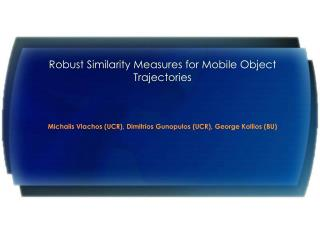# Robust Similarity Measures for Mobile Object Trajectories - PowerPoint PPT PresentationDownload PresentationRobust Similarity Measures for Mobile Object Trajectories

Download Presentation## Robust Similarity Measures for Mobile Object Trajectories

- - - - - - - - - - - - - - - - - - - - - - - - - - - E N D - - - - - - - - - - - - - - - - - - - - - - - - - - -
##### Presentation Transcript

1. Robust Similarity Measures for Mobile Object Trajectories Michalis Vlachos (UCR), Dimitrios Gunopulos (UCR), George Kollios (BU) MDDS ‘02

2. Introduction Problem:Discover similar trajectories of moving objects Examples: • Features extracted from video-clips • Animal Mobility Experiments (GPS data) • Sign Language Recognition, etc.

3. Applications & Requirements Clustering Classification What do we need? • Similarity Measure (robust to noise) • Indexing Scheme MDDS ‘02

4. Outline • Related Work (Euclidean Distance, Time Warping) • Extension of LCSS model to 2d trajectories • Algorithms for Computing the new similarity model • Flexible Sigmoidal Matching • Comparison with Lp-Norms and DTW distance • Conclusions, Future Work MDDS ‘02

5. Related Work – Euclidean Distance • Disadvantages • Small Robustness to outliers • Sensitive to time axis displacement • Does not support variable lengths • Lp–Norm: LP=(Σ(xi-yi)p)1/p • L2: Euclidean Distance • L1: Manhattan Distance MDDS ‘02

6. Related Work – DTW • Time Warping • Allows stretching in time axis • Difficult Indexing • Disadvantages • Computationally intensive, O(n*m) • Has to match ALL elements MDDS ‘02

7. Requirements for new Similarity Model (1) We need to address the following issues: • Different Sampling Rates or Different Speeds MDDS ‘02

8. Requirements for new Similarity Model (2) We need to address the following issues: • Similar Motions in different space Regions MDDS ‘02

9. Requirements for new Similarity Model (3) We need to address the following issues: • Outliers Non Recoverable Part Noise Everywhere Random Peaks • Different Lengths MDDS ‘02

10. Longest Common Subsequence (LCSS) • Dynamic Programming Solution • Arithmetic Example: • t1=[0, 4, 6, 8, 7, 4, 6, 5, 6, 4, 6] • t2=[0, 3, 4, 6, 7, 6, 3, 6, 4, 6 ] MDDS ‘02

11. Extending LCSS (1) We extend the LCSS to 2-dimensions and add more flexibility: Similarity of 2 seq/s with length n & m: MDDS ‘02

12. Extending LCSS – Example 2ε • Rigid matching • Points marginally outside matching region are ignored • Set parameter epsilon MDDS ‘02

13. Sigmoidal Matching MDDS ‘02

14. Computation Algorithms for new models (S1) • Computing Similarity S1 Lemma 1:Given two trajectories A and B, with |A|=n and |B|=m, we can find the SigmoidSimδ(Α,Β) in O(δ(n+m)) time MDDS ‘02

15. f(B) c d Extending LCSS (2) • S1 cannot detect parallel movements, Time B Y X • So, we define S2: • S2 can detect parallel movements • Better accuracy than simple normalization • Distance D1= 1-S1 & distance D2 = 1-S2 MDDS ‘02

16. 6 6 5 5 4 4 3 3 2 2 1 1 Exact Algorithm for similarity function S2 For trajectories A, B with length n we want to find: • translation fc,dthat maximizes SigmoidSim between A and fc,d (B) • Not infinite translations. • Each dimension separately • A translation in 1D: fc(bi) = bi + c (line with slope 1) • fc(bi) will allow bi to be matched to all aj: |i-j|<δ & ai-ε ≤ fc(bi) ≤(bi, aj+ε) • Transform into a stabbing problem Translations : O(δ2n2) LCSS : O(δn) Total : O(δ3n3) y=x+2 y=x MDDS ‘02

17. Approximate Algorithm for similarity function S2 A translation corresponds to a line fc(x) = x+c. • Sort translations by c  THEY DIFFER IN HOW MANY SEGMENTS? • If we can afford to be within βof max(Sim) we can afford to lose βn elements • Don’t take all translations  we can examine every βntranslations each time • So, if we examine every βn, we lose at mostβnelements (1D) • So, for 2D, we can skip every βn/2 translations MDDS ‘02

18. Example: • |A| = |B| = 1000, δ=2, β=0.04=>b=0.04*1000/2=20 • total # translations: 2δn = 4000, {-100, -98, -95,…,-30, -10, 0,…, 0.1, 2, 3.3, ..} • # translations we consider: 2δn/b = 200;in 2d 400 times less translations Approximate Algorithm for similarity function S2 Theorem: Given two trajectories A and B, with |A| = n and |B|=n, and a constant 0<β<1, we can find an approximation AS2δ,β(A,B) of the similarity S2(δ,ε,A,B) such that S2(δ,ε,A,B) - AS2δ,β(A,B) < β in O(nδ3/ β2) time. MDDS ‘02

19. Approximate Algorithm for similarity function S2 (cont/d) Theorem: Given two trajectories A and B, with |A| = n and |B|=n, and a constant 0<β<1, we can find an approximation AS2δ,β(A,B) of the similarity S2(ε,A,B) such that S2(δ,ε,A,B) - AS2δ,β(A,B) < aβ in O(nδ3/ β2) time, for a constant a. MDDS ‘02

20. Clustering Accuracy Datasets: • MobileLong • MobileShort • MobileShort + Noise Test clustering accuracy using Hierarchical Clustering C1 C2 C3 C4 C5 MDDS ‘02

21. DTW SIGMOIDSIM Clustering Accuracy • Lp–Norm: LP=(Σ(xi-yi)p)1/p • DTW = Lp + min((Head(A), B), (A,Head(B)), (Head(A), Head(B))) • SigmoidSim without translation MDDS ‘02

22. Clustering Accuracy (MobileLong) • Number of Correct Clusterings out of 10 MDDS ‘02

23. Clustering Accuracy (MobileShort) • Number of Correct Clusterings out of 21 MDDS ‘02

24. Clustering Accuracy (MobileShort + Noise) • Number of Correct Clusterings out of 21 MDDS ‘02

25. Conclusions, Future Work • Sigmoid Similarity provides best results under noise • Optimal translation can be found • Approximate solutions with provable performance bounds FUTURE WORK • Improve LCSS performance • Trajectory Segmentation • Add Scaling & Rotation MDDS ‘02

26. MDDS ‘02